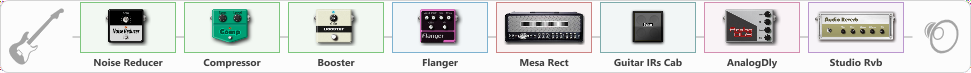# truss

Discussion in 'ToneLib-GFX presets' started by truss van halen, Jun 4, 2020.

1. ### truss van halenWell-Known Member

truss

Preset name: Mirror truss

Effects chain:Effect: "Noise Reducer" (Dynamics / Filter), active - "yes"
{
"Sens" = 100
"Mode" = Hard
}

Effect: "Compressor" (Dynamics / Filter), active - "yes"
{
"Sense" = 39
"Level" = 42
}

Effect: "Booster" (Dynamics / Filter), active - "yes"
{
"Gain" = 63
}

Effect: "Flanger" (Modulation / Sfx), active - "yes"
{
"Speed" = 1.4
"Depth" = 26
"Reso" = 13
"Center" = 7.7
"Offset" = 2.5
"Mix" = 75
"Reset" = On
}

Effect: "Mesa Rect" (Amp simulators), active - "yes"
{
"Gain" = 18
"Bass" = 23
"Middle" = 100
"Treble" = 75
"Presence" = 69
"Master" = 76
"Level (dB)" = 0
}

Effect: "Guitar IRs Cab" (Cabinets), active - "yes"
{
"Model" = Mesa Boogie Mk3 (1x12")
"Mic Position" = Center
"Mic Distance" = Near
"Low Cut (Hz)" = 192
"Hi Cut (kHz)" = 12.5
"Mix" = 100
"Level (dB)" = 0
}

Effect: "AnalogDly" (Delay), active - "yes"
{
"Time" = 396
"Feedback" = 54
"Tone" = 74
"Mix" = 60
}

Effect: "Studio Rvb" (Reverb), active - "yes"
{
"Time" = 6.7
"PreDelay" = 13
"LoDamp" = 0
"HiDamp" = 10
"Mix" = 60
}

Note: You will need to download and install the ToneLib-GFX software to use the preset.

#### Attached Files:

• ###### truss.tlgfx
File size:
743 bytes
Views:
2,851
Alexey Borovkov likes this.